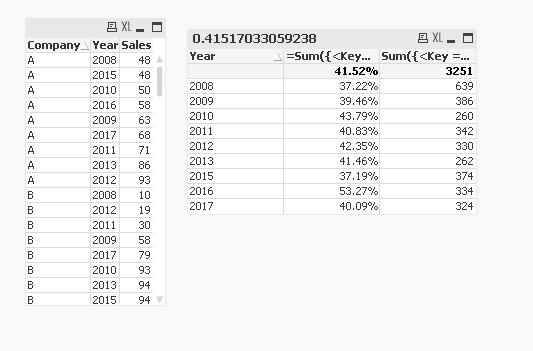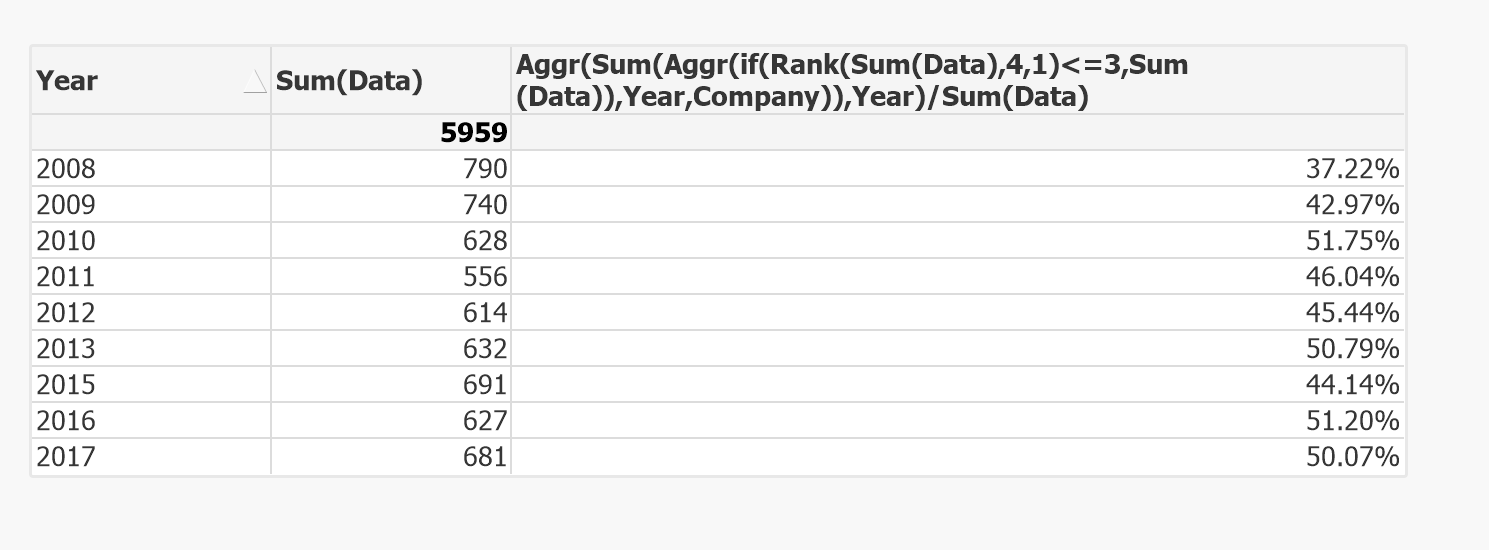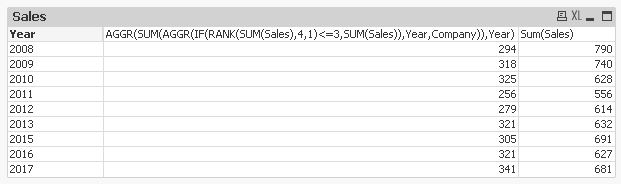New to QlikView

Discussion board where members can get started with QlikView.

Announcements
QlikWorld 2020: Join us May 11 - 14, 2020 in Phoenix, AZ. Register early and save \$400. Learn More
HighlightedNot applicable

Sum largest values each year. Rank, Sum & Aggr?

I'm trying to make a graph which shows the sum of the 3 largest values each year as a percentage of the sum of all values each year. I've tried to use rank, sum and aggr, but it does not work for me. Can someone guide me in the right direction by providing me with a link to a thread where this is discussed (I have not found one that's appropriate, but perhaps you will) or by helping me directly? Pleas find attached example of input data to QV.

1 Solution

Accepted SolutionsMVP

Re: Sum largest values each year. Rank, Sum & Aggr?

Try this

=Sum({<Key = {"=Aggr(Rank(Sum(Sales)), Year, Company) < 4"}>} Sales)/Sum(Sales)

Where Key is created in the script

Table:

CrossTable(Year, Sales)

,

,

,

,

,

,

,

,



FROM

(ooxml, embedded labels, table is QV);

FinalTable:

Company&Year as Key

Resident Table;

DROP Table Table;7 RepliesMVP

Re: Sum largest values each year. Rank, Sum & Aggr?

What would be the expected output based on the sample attached?MVP

Re: Sum largest values each year. Rank, Sum & Aggr?

Also, do you have years in different columns or do you have an year field?

Honored Contributor III

Re: Sum largest values each year. Rank, Sum & Aggr?

maybe you can check if this is correct -Not applicable

Re: Sum largest values each year. Rank, Sum & Aggr?

Almost. I have had this problem before. It seems as if the formula sums more values than the three biggest values... The first year is correct, but the second is not correct when I compare to my excel sheet.MVP

Re: Sum largest values each year. Rank, Sum & Aggr?

Company A repeats twice, is this by mistake?MVP

Re: Sum largest values each year. Rank, Sum & Aggr?

Try this

=Sum({<Key = {"=Aggr(Rank(Sum(Sales)), Year, Company) < 4"}>} Sales)/Sum(Sales)

Where Key is created in the script

Table:

CrossTable(Year, Sales)

,

,

,

,

,

,

,

,



FROM

(ooxml, embedded labels, table is QV);

FinalTable:

Company&Year as Key

Resident Table;

DROP Table Table;Honored Contributor III

Re: Sum largest values each year. Rank, Sum & Aggr?

The problem is only due to two rows for Company A, it has aggregated both values and that is why it is making into first three ranks after first row. First row addition rank is not making into first 3 show it is showing correct value. having two rows for Company A is right behavior or its a data issue?